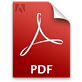# Mathematical Logic and Philosophy

## Description:

Computers are changing the way we do mathematics, as well as introducing new research agendas. Computational methods in mathematics, including symbolic and numerical computation and simulation, are by now familiar. These lectures will explore the way that "formal methods," based on formal languages and logic, can contribute to mathematics as well. In the 19th century, George Boole argued that if we take mathematics to be the science of calculation, then symbolic logic should be viewed as a branch of mathematics: just as number theory and analysis provide means to calculate with numbers, logic provides means to calculate with propositions. Computers are, indeed, good at calculating with propositions, and there are at least two ways that this can be mathematically useful: first, in the discovery of new proofs, and, second, in verifying the correctness of existing ones. The first goal generally falls under the ambit of "automated theorem proving" and the second falls under the ambit of "interactive theorem proving." There is no sharp distinction between these two fields, however, and the line between them is becoming increasingly blurry. In these lectures, I will provide an overview of both fields and the interactions between them, and speculate as to the roles they can play in mainstream mathematics. I will aim to make the lectures accessible to a broad audience. The first lecture will provide a self-contained overview. The remaining lectures are for the most part independent of one another, and will not rely on the first lecture.

## Lectures 1 and 2 (Duration: 2 hours 1 minute)DOWNLOAD LECTURE 1 SLIDESDOWNLOAD LECTURE 2 SLIDES

## Lecture 3 (Duration: 1 hour 14 minutes)DOWNLOAD LECTURE 3 SLIDES

## Lecture 4 (Duration: 58 minutes 48 seconds)DOWNLOAD LECTURE 4 SLIDES